# Q3. . Suppose that joint probability function of X and Y is given by | 1/7,...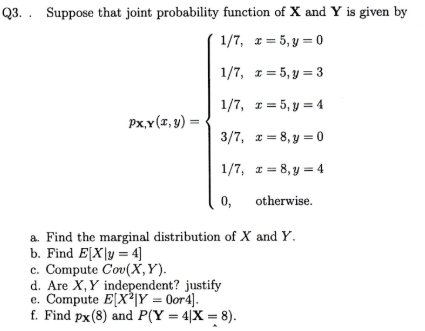Q3. . Suppose that joint probability function of X and Y is given by | 1/7, z = 5, y = 0 Px,y(, ) 0, otherwise. a. Find the marginal distribution of X and Y b. Find E(X|y = 4] c. Compute Cov(X, Y). d. Are X, Y independent? justify e. Compute E[XY0or4] f. Find px(8) and P(Y-4X-8).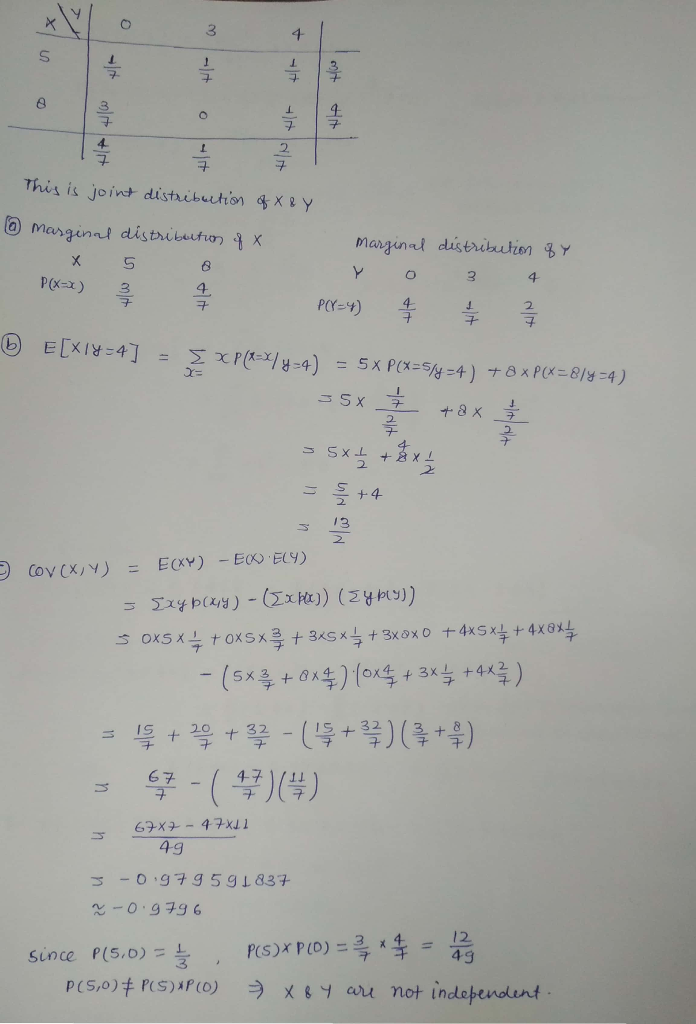#### Earn Coin

Coins can be redeemed for fabulous gifts.

Similar Homework Help Questions
• ### 1. Let the joint probability (mass) function of X and Y be given by the following:...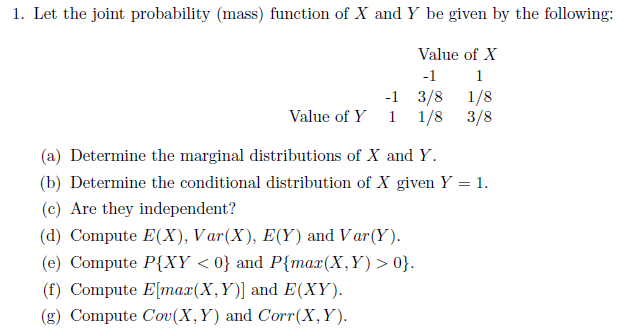1. Let the joint probability (mass) function of X and Y be given by the following: Value of X -1 -1 3/8 1/8 Value of Y1 1/8 3/8 (a) Determine the marginal (b) Determine the conditional distribution of X given Y (c) Are they independent? d) Compute E(X), Var(X), E(Y) and Var(Y). (e) Compute PXY <0) and Ptmax(X,Y) > 0 (f) Compute Elmax(X, Y)] and E(XY) (g) Compute Cov(X,Y) and Corr(X, Y) 1

• ### 3. The joint probability density function of X and Y is given by 2 if O< x S 2,0 < y, and x +ys1 ...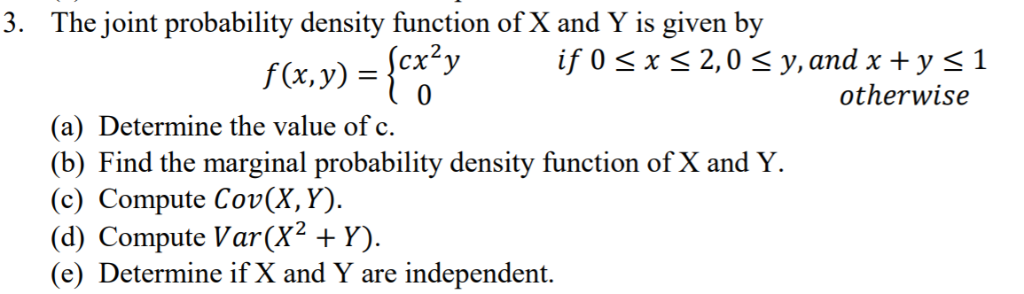Please do not copy, all the previous answers are wrong. 3. The joint probability density function of X and Y is given by 2 if O< x S 2,0 < y, and x +ys1 otherwise f(x,y) = 〉cry (a) Determine the value of c (b) Find the marginal probability density function of X and Y (c) Compute Cov(X, Y) (d) Compute Var(X2 Y) (e) Determine if X and Y are independent. 3. The joint probability density function of X and...

• ### 7. The random variables X and Y have joint probability density function f given by 1...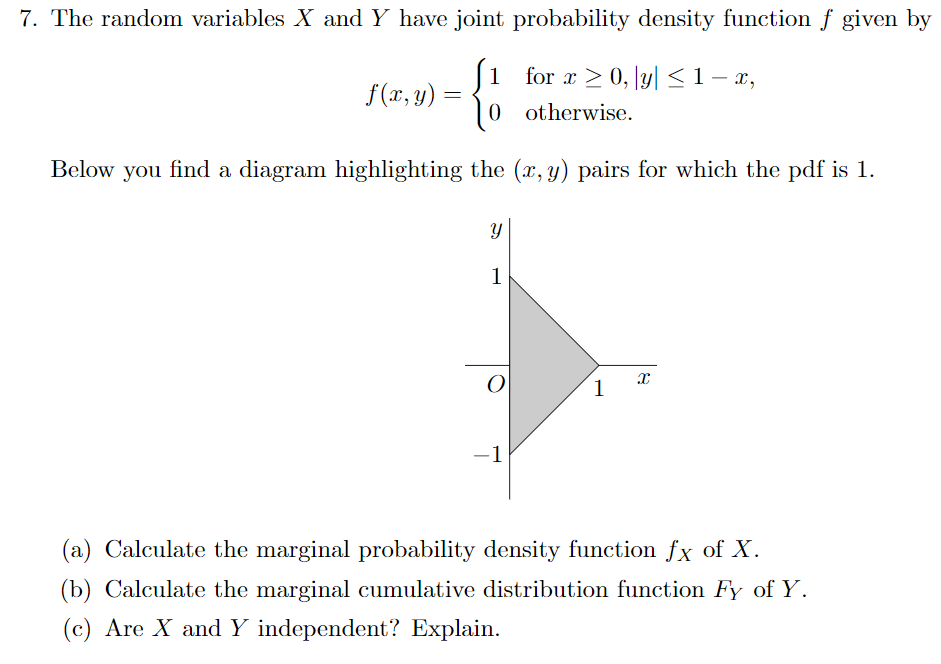7. The random variables X and Y have joint probability density function f given by 1 for x > 0, |y| 0 otherwise. 1-x, Below you find a diagram highlighting the (r, y) pairs for which the pdf is 1 (a) Calculate the marginal probability density function fx of X (b) Calculate the marginal cumulative distribution function Fy of Y (c) Are X and Y independent? Explain.

• ### 7. The random variables X and Y have joint probability density function f given by 1...7. The random variables X and Y have joint probability density function f given by 1 for x > 0, |y| 0 otherwise. 1-x, Below you find a diagram highlighting the (r, y) pairs for which the pdf is 1 (a) Calculate the marginal probability density function fx of X (b) Calculate the marginal cumulative distribution function Fy of Y (c) Are X and Y independent? Explain.

• ### 2. Let X and Y be continuous random variables with joint probability density function fx,y(x,y) 0, otherwise (a) Compute the value of k that will make f(x, y) a legitimate joint probability density f...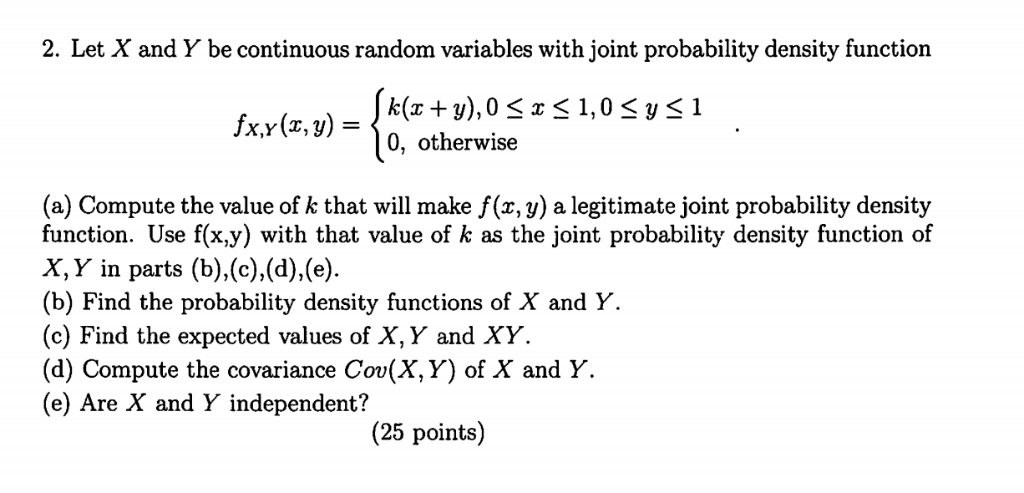2. Let X and Y be continuous random variables with joint probability density function fx,y(x,y) 0, otherwise (a) Compute the value of k that will make f(x, y) a legitimate joint probability density function. Use f(x.y) with that value of k as the joint probability density function of X, Y in parts (b),(c).(d),(e (b) Find the probability density functions of X and Y. (c) Find the expected values of X, Y and XY (d) Compute the covariance Cov(X,Y) of X...

• ### Questionl The random variable X and Y have the following joint probability mass function: 0.14 0.27...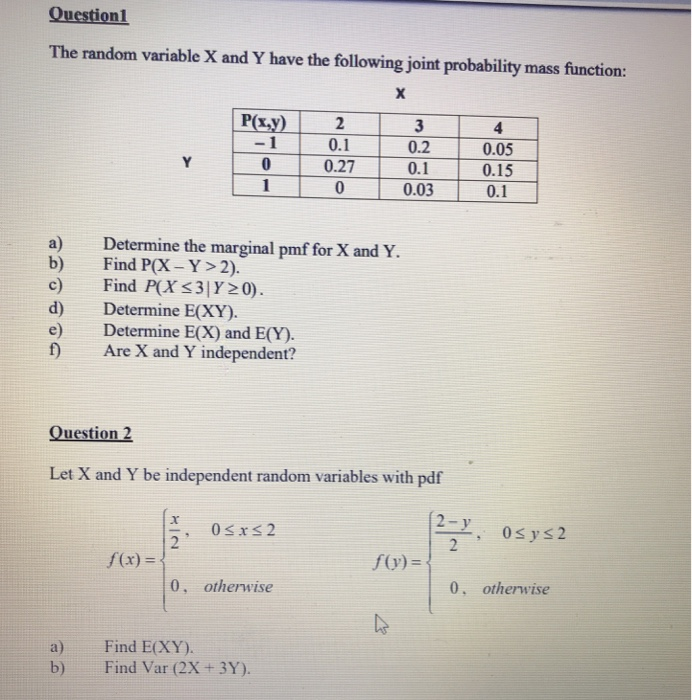Questionl The random variable X and Y have the following joint probability mass function: 0.14 0.27 0.2 0.1 0.03 0.15 0.1 a) Determine the b) Find P(X-Y>2). c) Find PX s3|Y20) d) Determine E(XY) e) Determine E(X) and E(Y). f) Are X and Y independent? marginal pmf for X and Y. Question 2 Let X and Y be independent random variables with pdf 2-y 0sxS 2 f(x)- f(p)- 0, otherwise 0, otherwise a) b) Find E(XY). Find Var (2X +...

• ### 1. Suppose you have two random variables, X and Y with joint distribution given by the...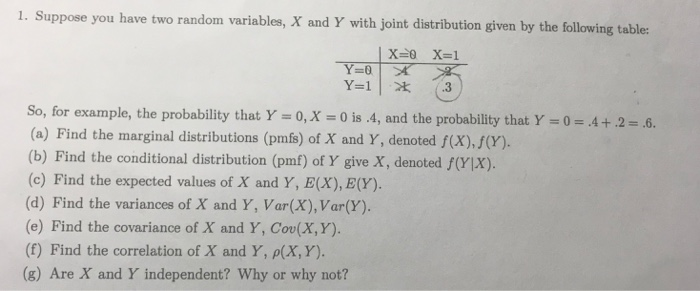1. Suppose you have two random variables, X and Y with joint distribution given by the following tables So, for example, the probability that Y o,x - 0 is 4, and the probability that Y (a) Find the marginal distributions (pmfs) of X and Y, denoted f(x),J(Y). (b) Find the conditional distribution (pmf) of Y give X, denoted f(YX). (c) Find the expected values of X and Y, EX), E(Y). (d) Find the variances of X and Y, Var(X),Var(Y). (e)...

• ### Q4. Suppose X is a random variables with possible values -1,1 and Y is another with...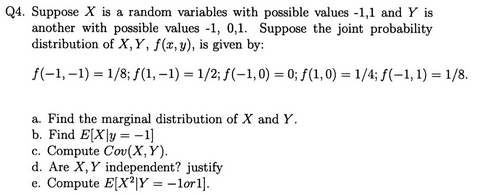Q4. Suppose X is a random variables with possible values -1,1 and Y is another with possible values -1, 0,1. Suppose the joint probability distribution of X, Y, f(x, y), is given by: a. Find the marginal distribution of X and Y. b. Find E[Xly1] c. Compute Cov(X, Y) d. Are X, Y independent? justify e. Compute E(XYorl

• ### 1. The joint probability density function (pdf) of X and Y is given by fxy(x, y)...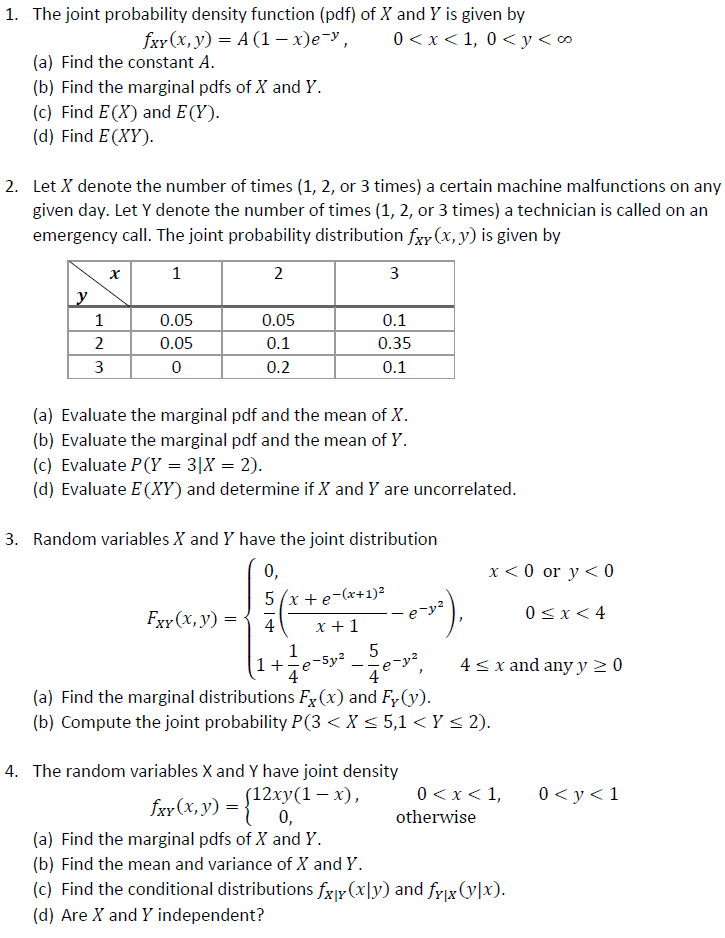1. The joint probability density function (pdf) of X and Y is given by fxy(x, y) = A (1 – xey, 0<x<1,0 < y < 0 (a) Find the constant A. (b) Find the marginal pdfs of X and Y. (c) Find E(X) and E(Y). (d) Find E(XY). 2. Let X denote the number of times (1, 2, or 3 times) a certain machine malfunctions on any given day. Let Y denote the number of times (1, 2, or 3...

• ### (20 points) Consider the following joint distribution of X and Y ㄨㄧㄚ 0 0.1 0.2 1 0.3 0.4 (a) Find the marginal distributions of X and Y. (i.e., Px(x) and Py()) (b) Find the conditional distributio...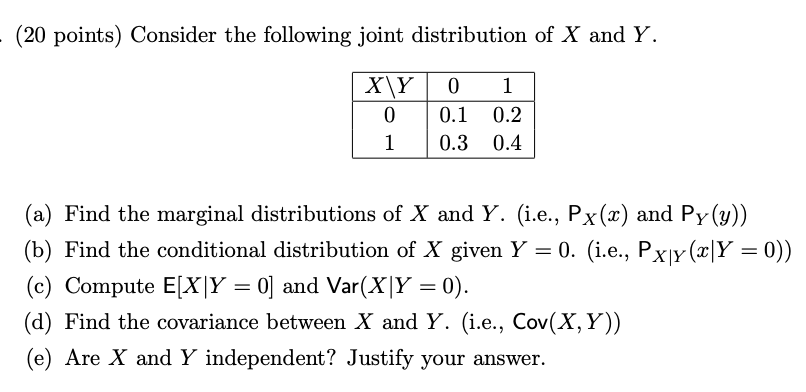(20 points) Consider the following joint distribution of X and Y ㄨㄧㄚ 0 0.1 0.2 1 0.3 0.4 (a) Find the marginal distributions of X and Y. (i.e., Px(x) and Py()) (b) Find the conditional distribution of X given Y-0. (i.e., Pxjy (xY-0)) (c) Compute EXIY-01 and Var(X)Y = 0). (d) Find the covariance between X and Y. (i.e., Cov(X, Y)) (e) Are X and Y independent? Justify your answer. (20 points) Consider the following joint distribution of X and...